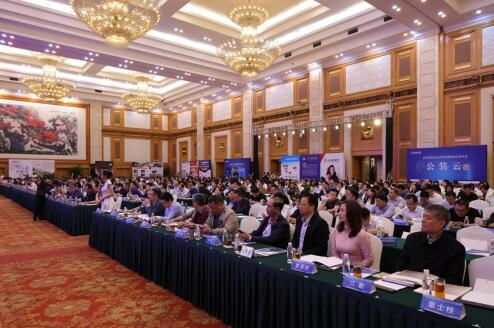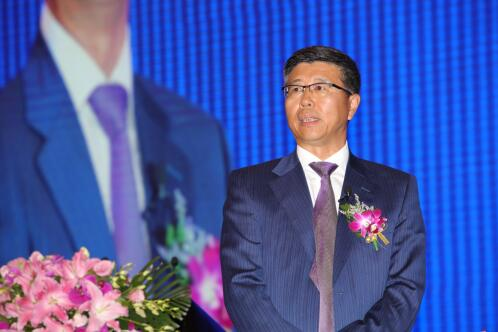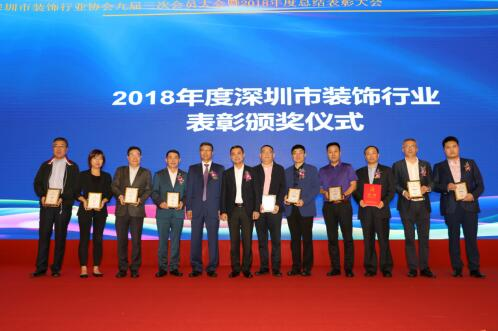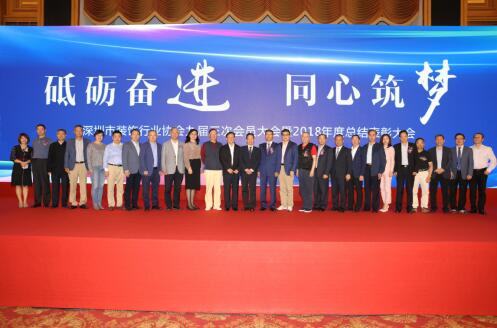|

# 深圳市装饰行业协会会员大会暨总结表彰大会召开

2019年3月28日下午，深圳市装饰行业协会九届三次会员大会暨2018年度总结表彰大会在深圳五洲宾馆召开。

中国建筑装饰协会名誉会长马挺贵、执行会长兼秘书长刘晓一，深圳市住房和建设局局长张学凡、副局长兼总工程师高尔剑、建筑市场与招投标监管处处长林霞，深圳市社会组织管理局副局长孙景明、副局长李文海等领导出席了会议。深圳市装饰行业协会会长高刚，深圳市装饰行业协会名誉会长、广田控股董事长叶远西，常务副会长庄志伟、常务副会长王彦波、副会长郑鑫、监事长潘育明，以及各装饰企业家及会员代表参加了会议。大会由深圳市装饰行业协会秘书长刘静主持。王彦波常务副会长作了题为《攻坚克难 行稳致远 同心筑梦 继往开来》的2018年工作总结报告，回顾了过去一年深圳装饰行业的成绩以及协会工作的完成情况。

高刚会长在会上作了题为《坚毅笃行谋发展 砥砺奋进新时代》的2019年度工作报告。报告指出，随着供给侧结构性改革深入推进，2019年深圳装饰行业应回归装饰主业，把握国家新旧动能转换带来产业结构调整的趋势，在稳增长、调结构、防风险的国家战略中寻找生机和希望。装饰行业一是抢抓粤港澳大湾区建设的历史机遇;二是推动高质量发展，推动优质优价，“让优秀的装饰资源”为深圳建设服务;三是加快推动装饰行业产业工人队伍培育建设;四是抓好行业党建，持续推进行业廉洁自律。潘育明监事长代表监事会作了2018年度监事会工作报告和协会财务工作报告，提请大会审议。

深圳市住房和建设局局长张学凡在大会上作了重要讲话，他说，深圳装饰业已经成为了中国装饰业最高水平的代表，这是我们深圳全体装饰人的荣耀，是深圳这座城市的骄傲，这些成就的取得，离不开装饰行业各企业、科研机构、专家学者的共同努力和不懈追求，离不开高刚会长以及历任会长带领下深圳装饰行业协会的创新开拓和辛勤付出。他希望，已经成为“金字招牌”的深圳装饰行业，要抓住粤港澳大湾区建设重大机遇，要按照“世界眼光、国际标准、中国特色、高点定位”，坚持科技创新引领，继续引领姿态，高位求进、实干争先，在新时代实现新作为。

深圳市社会组织管理局副局长李文海在致辞中高度肯定了深圳市装饰行业协会的工作成果，认为协会有效地联动政府和企业，有力地推动了深圳装饰行业的发展进步，充分发挥了管理者和服务者的价值。中国建筑装饰协会执行会长兼秘书长刘晓一讲话，他指出，深圳市装饰行业协会在反映企业诉求、行业自律和诚信建设、促进工程管理水平提高、推广应用先进技术以及提高行业人员素质等方面，做了大量富有成效的工作，很好地履行了协会职能，赢得了各界的认可和企业的拥护，他希望深圳装饰企业在新时期把握新方向，聚焦新战略，迈上新台阶，全面实现健康、长远、有序的跨越发展。

大会还审议通过了相关议案，圆满完成了各项议程，得到了广大会员单位的肯定和赞誉。大会举行了隆重的颁奖仪式。2018年度深圳市装饰行业“创新型人才”、“杰出贡献企业”、“十大优秀项目经理”、“十大青年设计师”以及“科技人才”、“明星工匠”、“科技创新成果”等奖项一一揭晓。通过对行业内表现突出的优秀个人、优秀企业予以表彰，并向社会宣传发布，甄选优秀的行业生产力资源，为深圳装饰行业发展服务，为深圳建设服务，持续助力全行业提升发展质量，向更加健康有序的新时期发展道路迈进。

参加大会的嘉宾还有：深圳市住建局原局长、深装协顾问邹国华，深圳市住建局原副局长、深装协顾问胡建文，深圳市住建局原处长、深装协顾问马遥之，天津市环境装饰协会会长徐林晞，广西建筑装饰协会会长庞耀国，安徽省建筑装饰协会副会长、合肥市建筑装饰协会会长陶余桐，重庆市建筑装饰协会秘书长韩波，广东省建筑业协会副秘书长甄祖玲女士，广东省建筑装饰材料行业协会、广东省建筑装饰设计协会常务秘书长曹爱群，深圳市建筑业协会秘书长彭迎祥，深圳市土木建筑学会秘书长刘福义、深圳市防水行业协会会长瞿培华，深圳市建筑门窗幕墙学会秘书长江勤，深圳大学教授蔡强，交通银行深圳分行海连支行行长雍波。(星文社报道)`声明：本文由入驻焦点开放平台的作者撰写，除焦点官方账号外，观点仅代表作者本人，不代表焦点立场错误信息举报电话： 400-099-0099，邮箱：jubao@vip.sohu.com，或点此进行意见反馈，或点此进行举报投诉。`A B C D E F G H J K L M N P Q R S T W X Y Z
A - B - C - D - E
• A
• 鞍山
• 安庆
• 安阳
• 安顺
• 安康
• 澳门
• B
• 北京
• 保定
• 包头
• 巴彦淖尔
• 本溪
• 蚌埠
• 亳州
• 滨州
• 北海
• 百色
• 巴中
• 毕节
• 保山
• 宝鸡
• 白银
• 巴州
• C
• 承德
• 沧州
• 长治
• 赤峰
• 朝阳
• 长春
• 常州
• 滁州
• 池州
• 长沙
• 常德
• 郴州
• 潮州
• 崇左
• 重庆
• 成都
• 楚雄
• 昌都
• 慈溪
• 常熟
• D
• 大同
• 大连
• 丹东
• 大庆
• 东营
• 德州
• 东莞
• 德阳
• 达州
• 大理
• 德宏
• 定西
• 儋州
• 东平
• E
• 鄂尔多斯
• 鄂州
• 恩施
F - G - H - I - J
• F
• 抚顺
• 阜新
• 阜阳
• 福州
• 抚州
• 佛山
• 防城港
• G
• 赣州
• 广州
• 桂林
• 贵港
• 广元
• 广安
• 贵阳
• 固原
• H
• 邯郸
• 衡水
• 呼和浩特
• 呼伦贝尔
• 葫芦岛
• 哈尔滨
• 黑河
• 淮安
• 杭州
• 湖州
• 合肥
• 淮南
• 淮北
• 黄山
• 菏泽
• 鹤壁
• 黄石
• 黄冈
• 衡阳
• 怀化
• 惠州
• 河源
• 贺州
• 河池
• 海口
• 红河
• 汉中
• 海东
• 怀来
• I
• J
• 晋中
• 锦州
• 吉林
• 鸡西
• 佳木斯
• 嘉兴
• 金华
• 景德镇
• 九江
• 吉安
• 济南
• 济宁
• 焦作
• 荆门
• 荆州
• 江门
• 揭阳
• 金昌
• 酒泉
• 嘉峪关
K - L - M - N - P
• K
• 开封
• 昆明
• 昆山
• L
• 廊坊
• 临汾
• 辽阳
• 连云港
• 丽水
• 六安
• 龙岩
• 莱芜
• 临沂
• 聊城
• 洛阳
• 漯河
• 娄底
• 柳州
• 来宾
• 泸州
• 乐山
• 六盘水
• 丽江
• 临沧
• 拉萨
• 林芝
• 兰州
• 陇南
• M
• 牡丹江
• 马鞍山
• 茂名
• 梅州
• 绵阳
• 眉山
• N
• 南京
• 南通
• 宁波
• 南平
• 宁德
• 南昌
• 南阳
• 南宁
• 内江
• 南充
• P
• 盘锦
• 莆田
• 平顶山
• 濮阳
• 攀枝花
• 普洱
• 平凉
Q - R - S - T - W
• Q
• 秦皇岛
• 齐齐哈尔
• 衢州
• 泉州
• 青岛
• 清远
• 钦州
• 黔南
• 曲靖
• 庆阳
• R
• 日照
• 日喀则
• S
• 石家庄
• 沈阳
• 双鸭山
• 绥化
• 上海
• 苏州
• 宿迁
• 绍兴
• 宿州
• 三明
• 上饶
• 三门峡
• 商丘
• 十堰
• 随州
• 邵阳
• 韶关
• 深圳
• 汕头
• 汕尾
• 三亚
• 三沙
• 遂宁
• 山南
• 商洛
• 石嘴山
• T
• 天津
• 唐山
• 太原
• 通辽
• 铁岭
• 泰州
• 台州
• 铜陵
• 泰安
• 铜仁
• 铜川
• 天水
• 天门
• W
• 乌海
• 乌兰察布
• 无锡
• 温州
• 芜湖
• 潍坊
• 威海
• 武汉
• 梧州
• 渭南
• 武威
• 吴忠
• 乌鲁木齐
X - Y - Z
• X
• 邢台
• 徐州
• 宣城
• 厦门
• 新乡
• 许昌
• 信阳
• 襄阳
• 孝感
• 咸宁
• 湘潭
• 湘西
• 西双版纳
• 西安
• 咸阳
• 西宁
• 仙桃
• 西昌
• Y
• 运城
• 营口
• 盐城
• 扬州
• 鹰潭
• 宜春
• 烟台
• 宜昌
• 岳阳
• 益阳
• 永州
• 阳江
• 云浮
• 玉林
• 宜宾
• 雅安
• 玉溪
• 延安
• 榆林
• 银川
• Z
• 张家口
• 镇江
• 舟山
• 漳州
• 淄博
• 枣庄
• 郑州
• 周口
• 驻马店
• 株洲
• 张家界
• 珠海
• 湛江
• 肇庆
• 中山
• 自贡
• 资阳
• 遵义
• 昭通
• 张掖
• 中卫

1室1厅1厨1卫1阳台

1
2
3
4
5

0
1
2

1

1

0
1
2
3报名成功，资料已提交审核A B C D E F G H J K L M N P Q R S T W X Y Z
A - B - C - D - E
• A
• 鞍山
• 安庆
• 安阳
• 安顺
• 安康
• 澳门
• B
• 北京
• 保定
• 包头
• 巴彦淖尔
• 本溪
• 蚌埠
• 亳州
• 滨州
• 北海
• 百色
• 巴中
• 毕节
• 保山
• 宝鸡
• 白银
• 巴州
• C
• 承德
• 沧州
• 长治
• 赤峰
• 朝阳
• 长春
• 常州
• 滁州
• 池州
• 长沙
• 常德
• 郴州
• 潮州
• 崇左
• 重庆
• 成都
• 楚雄
• 昌都
• 慈溪
• 常熟
• D
• 大同
• 大连
• 丹东
• 大庆
• 东营
• 德州
• 东莞
• 德阳
• 达州
• 大理
• 德宏
• 定西
• 儋州
• 东平
• E
• 鄂尔多斯
• 鄂州
• 恩施
F - G - H - I - J
• F
• 抚顺
• 阜新
• 阜阳
• 福州
• 抚州
• 佛山
• 防城港
• G
• 赣州
• 广州
• 桂林
• 贵港
• 广元
• 广安
• 贵阳
• 固原
• H
• 邯郸
• 衡水
• 呼和浩特
• 呼伦贝尔
• 葫芦岛
• 哈尔滨
• 黑河
• 淮安
• 杭州
• 湖州
• 合肥
• 淮南
• 淮北
• 黄山
• 菏泽
• 鹤壁
• 黄石
• 黄冈
• 衡阳
• 怀化
• 惠州
• 河源
• 贺州
• 河池
• 海口
• 红河
• 汉中
• 海东
• 怀来
• I
• J
• 晋中
• 锦州
• 吉林
• 鸡西
• 佳木斯
• 嘉兴
• 金华
• 景德镇
• 九江
• 吉安
• 济南
• 济宁
• 焦作
• 荆门
• 荆州
• 江门
• 揭阳
• 金昌
• 酒泉
• 嘉峪关
K - L - M - N - P
• K
• 开封
• 昆明
• 昆山
• L
• 廊坊
• 临汾
• 辽阳
• 连云港
• 丽水
• 六安
• 龙岩
• 莱芜
• 临沂
• 聊城
• 洛阳
• 漯河
• 娄底
• 柳州
• 来宾
• 泸州
• 乐山
• 六盘水
• 丽江
• 临沧
• 拉萨
• 林芝
• 兰州
• 陇南
• M
• 牡丹江
• 马鞍山
• 茂名
• 梅州
• 绵阳
• 眉山
• N
• 南京
• 南通
• 宁波
• 南平
• 宁德
• 南昌
• 南阳
• 南宁
• 内江
• 南充
• P
• 盘锦
• 莆田
• 平顶山
• 濮阳
• 攀枝花
• 普洱
• 平凉
Q - R - S - T - W
• Q
• 秦皇岛
• 齐齐哈尔
• 衢州
• 泉州
• 青岛
• 清远
• 钦州
• 黔南
• 曲靖
• 庆阳
• R
• 日照
• 日喀则
• S
• 石家庄
• 沈阳
• 双鸭山
• 绥化
• 上海
• 苏州
• 宿迁
• 绍兴
• 宿州
• 三明
• 上饶
• 三门峡
• 商丘
• 十堰
• 随州
• 邵阳
• 韶关
• 深圳
• 汕头
• 汕尾
• 三亚
• 三沙
• 遂宁
• 山南
• 商洛
• 石嘴山
• T
• 天津
• 唐山
• 太原
• 通辽
• 铁岭
• 泰州
• 台州
• 铜陵
• 泰安
• 铜仁
• 铜川
• 天水
• 天门
• W
• 乌海
• 乌兰察布
• 无锡
• 温州
• 芜湖
• 潍坊
• 威海
• 武汉
• 梧州
• 渭南
• 武威
• 吴忠
• 乌鲁木齐
X - Y - Z
• X
• 邢台
• 徐州
• 宣城
• 厦门
• 新乡
• 许昌
• 信阳
• 襄阳
• 孝感
• 咸宁
• 湘潭
• 湘西
• 西双版纳
• 西安
• 咸阳
• 西宁
• 仙桃
• 西昌
• Y
• 运城
• 营口
• 盐城
• 扬州
• 鹰潭
• 宜春
• 烟台
• 宜昌
• 岳阳
• 益阳
• 永州
• 阳江
• 云浮
• 玉林
• 宜宾
• 雅安
• 玉溪
• 延安
• 榆林
• 银川
• Z
• 张家口
• 镇江
• 舟山
• 漳州
• 淄博
• 枣庄
• 郑州
• 周口
• 驻马店
• 株洲
• 张家界
• 珠海
• 湛江
• 肇庆
• 中山
• 自贡
• 资阳
• 遵义
• 昭通
• 张掖
• 中卫• 手机• 分享
• 设计
免费设计
• 计算器
装修计算器
• 入驻
合作入驻
• 联系
联系我们
• 置顶
返回顶部